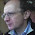## Friday, 13 July 2012

### .Net Tips , C# Tip : Check file contents is changed using calculate and comparing hash code of a file using C# Examples and VB.Net Examples

Hash code of a file is useful for check that file contents is changed over the time or not. First time you calculate hash code of a file and store , after some time period you again calculate hash code of a file and compare with stored hash code, If hash code is changed that means contents of the file is changed.

You can create a cryptographic hash code of the file using the ComputeHash method of the System.Security.Cryptography.HashAlgorithm class. Store calculated hash code for future comparison against newly calculated hash code of the same file. Hashing algorithm will generate a very different hash code even if the file has been changed slightly, and the chances of two different files resulting in the same hash code are very small.

Here is example of this.
In this example we are calculating hash code of a file.
We are calculating hash code using "SHA1" Hashing algorithm.
If you calculate hash code of same file using another hashing algorithm like "MD5" or "RIPEMD-160" it will produce different hash code.

C# Example :
```        string strFile = Server.MapPath("TextFile.txt");
using (HashAlgorithm objHashAlg = HashAlgorithm.Create("SHA1"))
{

using (Stream objFile = new FileStream(strFile, FileMode.Open, FileAccess.Read))
{
// Calculate the hash code of the file.
byte[] objHash = objHashAlg.ComputeHash(objFile);

Response.Write("<b>Calculated Hash Code :</b> "+ BitConverter.ToString(objHash));
}

}```

VB.net Example :
```        Dim strFile As String = Server.MapPath("TextFile.txt")
Using objHashAlg As HashAlgorithm = HashAlgorithm.Create("SHA1")

Using objFile As Stream = New FileStream(strFile, FileMode.Open, FileAccess.Read)
' Calculate the hash code of the file.
Dim objHash As Byte() = objHashAlg.ComputeHash(objFile)

Response.Write("<b>Calculated Hash Code :</b> " & BitConverter.ToString(objHash))

End Using
End Using```

Output :
(To view original image , click on image)

This is very useful .Net Tips.

Note : Give Us your valuable feedback in comments. Give your suggestions in this article so we can update our articles accordingly that.

1.1.